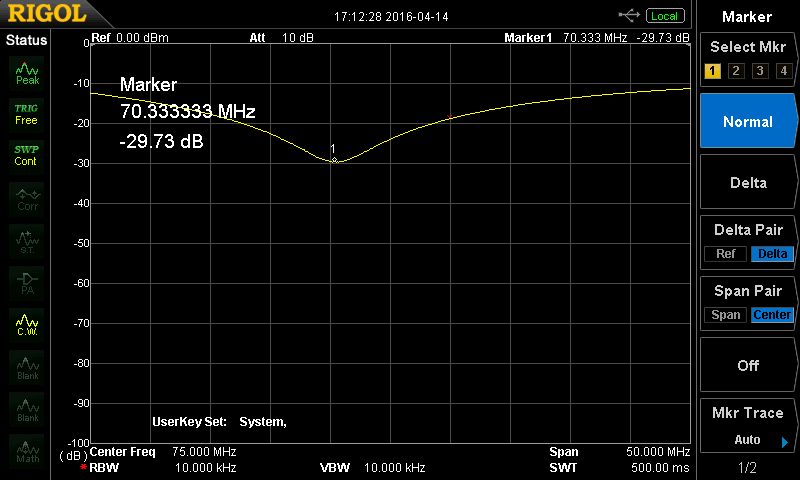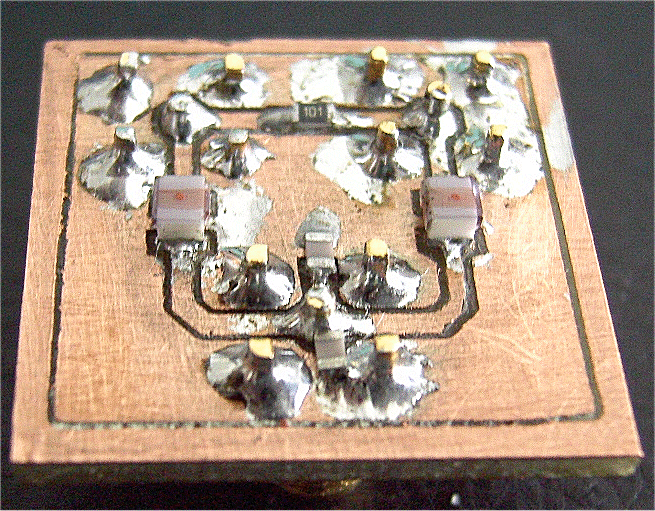### Calculating the Values

The values shown are for a 70MHz center frequency

Lz = SQRT(2) x Zo where Zo is the system impedance

L = Lz / 2x Pi x f (H) where f is the center frequency

C = 1 / (2 x Pi x f)2 x L (F)

C1 = 2 x C

R = 2 x Zo ohms

### Test results for the board I built### Insertion Loss

I have produced a PCB for the Splitter which may be of some use to you. The resistor and capacitor are 0805 and the inductors are Coilcraft 1008CS. The SMA's or SMB's are PCB mount. The PCB is double sided with a ground plane on the TOP. The track side is shown below. I have added the Gerber files for the track side and drilling.